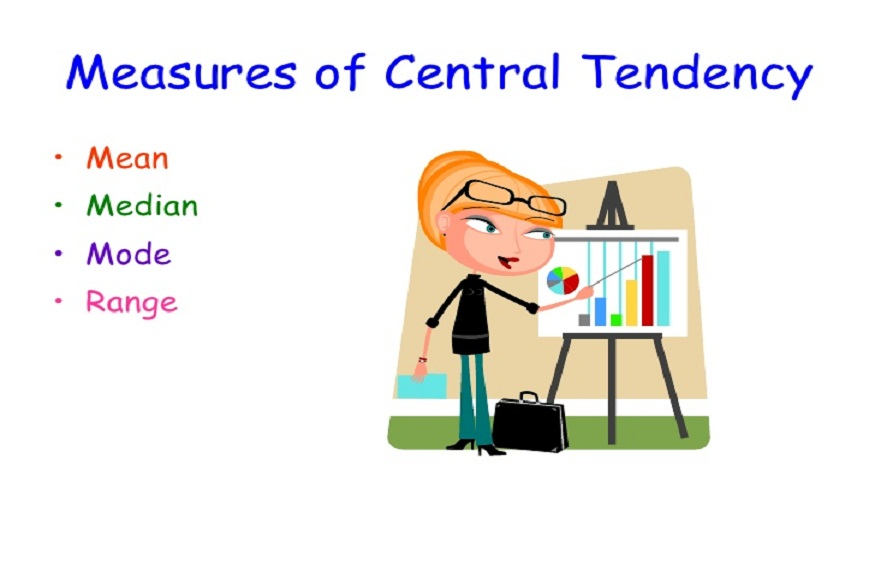# Measures of Central Tendency in Real Life586 Views

Statistics is a mathematical discipline that deals with the collection, classification, analysis, interpretation and presentation of data. Different types of statistical measures are computed on this data to draw some conclusions for analysing the real-life scenarios. Two major and important statistical methods used in data analysis are descriptive statistics and inferential statistics. We know that descriptive statistics review data from a sample with the help of indexes such as the mean or standard deviation. In contrast, inferential statistics help in concluding data that are subjected to random variation.

Measures of central tendency are generally used for finding the mid-value of given data sets. The three measures of central tendency in statistics include mean median and mode. All these three measures are used for computing the mid data value. The meaning of mean is given mathematically as the ratio of the sum of data values and the number of data values. This is the most commonly used statistic in real-life situations. For example, the mean daily wage (assuming that the wage of a worker varies day by day) of a worker can be computed by adding the daily wages and dividing it with the number of days.

Let us consider a situation where we need to make use of the statistical median. Generally, we may observe various income ranges and try to identify the group into which a specific person’s income belongs. Mean will not give an accurate value when we deal with the sample of people in a locality since the data being collected here may be open-ended or closed. When the last class being open-ended of the class greater than a certain number, say x. Here, it is not conceivable to compute the mean income. Hence, the median will be considered to get the required result from the collected data.

From the above example, we can say that the median is used when the distribution of the earnings provided. This is also used in sports to calculate the height of players in a particular team. In the economy, it helps in identifying the poverty line. Another important measure of central tendency is the mode. The mode is used to find the most frequent data value in the given set. This measure is used to identify the famous brand used by the customers for different categories of products such as clothes, beverages, beauty products and so on. Whichever the measure is used, all these three measures intended to find the average of the data. Also, we know that average and mean are computed similarly.

The measures of central tendency are used in other situations such as: finding the mean marks of the students in a class, in industries to identify whether the benefits of the employees are continued properly or not. Also, in assisting a family in balancing their expenses and income. Applications of statistics and its measures are not limited to only find the mean and averages of data provided for a particular situation but also applied in the experimental setting of samples from the population. Thus, different meaningful conclusions can be drawn from the samples by setting various suitable hypotheses for different situations.